Hypothesis Testing | IFT World
IFT Notes for Level I CFA® Program
IFT Notes for Level I CFA® Program

# Part 1

## 1. Introduction

Hypothesis testing is the process of making judgments about a larger group (a population) on the basis of observing a smaller group (a sample). The results of such a test then help us evaluate whether our hypothesis is true or false.

For example, let’s say you are a researcher and you believe that the average return on all Asian stocks was greater than 2%. To test this belief, you can draw samples from a population of all Asian stocks and employ hypothesis testing procedures. The results of this test can tell you if your belief is statistically valid.

In this reading we will look at hypothesis tests concerning the mean, variance and correlation.

## 2. The Process of Hypothesis Testing

A hypothesis is defined as a statement about one or more populations. In order to test a hypothesis, we follow these steps:

1. State the hypothesis.
2. Identify the appropriate test statistic and its probability distribution.
3. Specify the significance level.
4. State the decision rule.
5. Collect data and calculate the test statistic.
6. Make a decision.

### 2.1 Stating the Hypotheses

For each hypothesis test, we always state two hypotheses: the null hypothesis (H0) and the alternative hypothesis (Ha).

Null hypothesis (H0): It is the hypothesis that the researcher wants to reject.

Alternative hypothesis (Ha): It is the hypothesis that the researcher wants to prove. If the null hypothesis is rejected then the alternative hypothesis is considered valid.

Suppose you are a researcher and believe that the average return on all Asian stocks was greater than 2%. In this case, you are making a statement about the population mean (µ) of all Asian stocks.

For this example, the null and alternative hypotheses are:

H0: µ ≤ 2%

Ha: µ > 2%

(The value 2% is known as µ0, the hypothesized value of the population mean.)

Instructor’s Note:

An easy way to differentiate between the two hypotheses is to remember that the null hypothesis always contains some form of the equal sign.

### 2.2 Two-Sided vs. One-Sided Hypotheses

The alternative hypothesis can be one-sided or two-sided depending on the proposition being tested.  A one-sided test is also called a one-tailed test, and a two-sided test is also called a two-tailed test.

If we want to determine whether the estimated value of a population parameter is less than (or greater than) a hypothesized value we use a one-tailed test. However, if we want to determine whether the estimated value of a population parameter is different than a hypothesized value, we use a two tailed test.

Two-sided test

Suppose we want to test

if the population mean is equal to 2%. The null and alternative hypothesis can be expressed as:

H0: µ = 2%

Ha: µ ≠ 2%

Since the alternative hypothesis contains a ≠ sign this is a two-sided test.

One-sided test (right side): Suppose we want to test if the population mean is greater than 2%. The null and alternative hypothesis can be expressed as:

H0: µ ≤ 2%

Ha: µ > 2%

Since the alternative hypothesis contains a > sign this is a one-sided test, and we are interested in the right side.

Instructor’s Note

The sign in the alternative hypothesis points to the direction of the tail that we should use in our test. Since in our example the alternative hypothesis has a ‘>’ sign it points to the right, therefore we are interested in the right tail.

One-sided test (left side): Suppose we want to test if the population mean is greater than 2%. The null and alternative hypothesis can be expressed as:

H0: µ ≥ 2%

Ha: µ < 2%

Since the alternative hypothesis contains a < sign this is a one-sided test, and we are interested in the left side.

### 2.3 Selecting the Appropriate Hypotheses

The easiest approach is to specify the alternative hypothesis first and then specify the null. Using a ‘<’ or ‘>’ sign in the alternative hypothesis instead of a  ‘≠’ sign reflects that belief of the researcher more strongly. However, a researcher may sometimes select a two-sided test to depict neutrality in his beliefs.

## 3. Identify the Appropriate Test Statistic

### 3.1 Test Statistics

A test statistic is calculated from sample data and is compared to a critical value to decide whether or not we can reject the null hypothesis. The test statistic that should be used depends on what we are testing. For example, the test statistic for the test of a population mean is calculated as:

test statistic =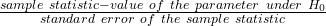=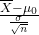Continuing with our Asian stocks example, suppose we want to test if the population mean is greater than a particular hypothesized value. We draw 36 observations and get a sample mean of 4. We are also told that the standard deviation of the population is 4. If the hypothesized value of the population mean is 2, the test statistic is calculated as:

test statistic =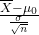=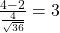However, if the hypothesized value of the population mean is 0, then the test statistic is calculated as:

test statistic ==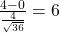### 3.2 Identifying the Distribution of the Test Statistic

Exhibit 4 from the curriculum shows which test-statistics should be used depending on what we want to test and their corresponding distributions.

Instructor’s Note: You will understand this table better, after you finish reading the remaining sections.

 What We Want to Test Test Statistic Probability Distribution of the Statistic Degrees of Freedom Test of a single mean t =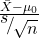t-Distributed n − 1 Test of the difference in means t=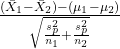t-Distributed n1 + n2 − 2 Test of the mean of differences t =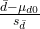t-Distributed n − 1 Test of a single variance x2 =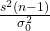Chi-square distributed n − 1 Test of the difference in variances F =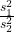F-distributed n1 − 1, n2 − 1 Test of a correlation t =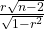t-Distributed n − 2 Test of independence (categorical data) x2 =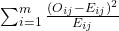Chi-square distributed (r − 1)(c − 1)